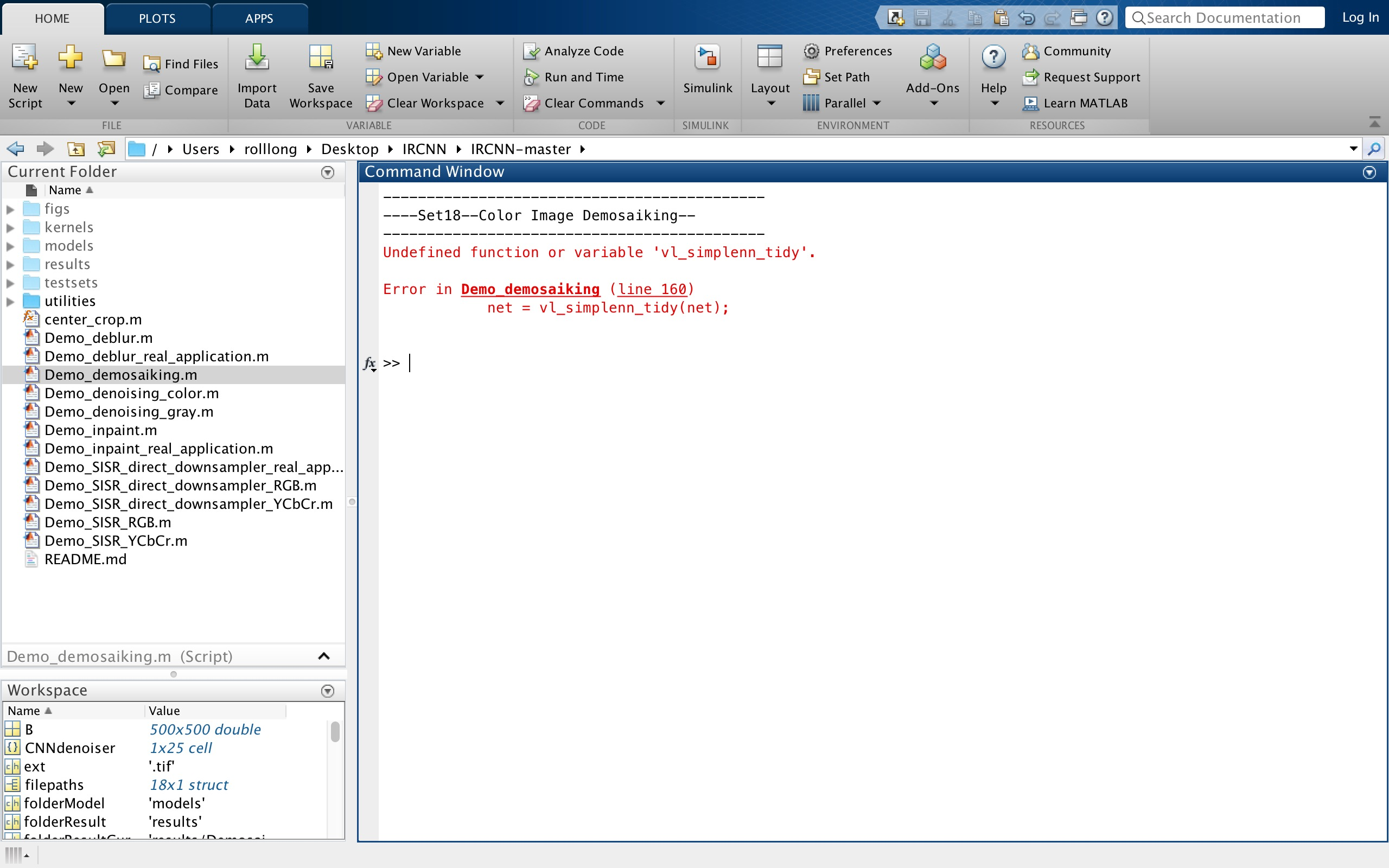matlab使用别人的代码的时候出现找不到函数的问题 40C

https://github.com/cszn/IRCNN`````` clear; clc;

imageSets    = {'Set18','Set24'}; % testing dataset
setTest      = imageSets(1); % select the dataset

useGPU       = 0;

folderTest   = 'testsets';
folderResult = 'results';
folderModel  = 'models';
if ~exist(folderResult,'file')
mkdir(folderResult);
end
setTestCur = cell2mat(setTest(1));
disp('--------------------------------------------');
disp(['----',setTestCur,'--Color Image Demosaiking--']);
disp('--------------------------------------------');
folderTestCur = fullfile(folderTest,setTestCur);

% folder to store results
folderResultCur = fullfile(folderResult, ['Demosaik_',setTestCur]);
if ~exist(folderResultCur,'file')
mkdir(folderResultCur);
end

%% Noise level
noiselevel = 0; % default; noiselevel = 10; Isigma     = 10/255; Msigma     = 8;

%% parameter setting in HQS (tune the following parameters to obtain the best results)
%% -------------------important!------------------
% Parameter settings of IRCNN
% (1) image noise level: Isigma
Isigma     = 0.5/255; % default 0.5/255 for noise-free image, ****** from interval [1/255, 20/255] ******; e.g., 1/255, 2.55/255, 7/255, 11/255
% (2) noise level of the last denoiser: Msigma
Msigma     = 2; % default 2 for noise-free image, ****** from {1 2 3 4 5 7 9 11 13 15} ******
%--------------------------------------------------------

%% default parameter setting in HQS
totalIter   = 30; % default 30
lamda       = (Isigma^2)/3; % default 3, ****** from {1 2 3 4} ******
modelSigma1 = 49; % default 49
modelSigmaS = logspace(log10(modelSigma1),log10(Msigma),totalIter);
rho         = Isigma^2/((modelSigma1/255)^2);

ns          = min(25,max(ceil(modelSigmaS/2),1));
ns          = [ns(1)-1,ns];

ext                 =  {'*.jpg','*.png','*.bmp','*.tif'};
filepaths           =  [];
for i = 1 : length(ext)
filepaths = cat(1,filepaths,dir(fullfile(folderTestCur, ext{i})));
end

PSNRs = zeros(1,length(filepaths));
SSIMs = zeros(1,length(filepaths));

for i = 1 : length(filepaths)

[~, Iname, ext] = fileparts(filepaths(i).name);
label = im2single(label);

[B, y, mask] = mosaic_bayer(label, 'grbg', noiselevel);
y    = single(y);
z = linearlcc(B, 0);
z =  single(z);

z0          = z;
if useGPU
z       = gpuArray(z);
y       = gpuArray(y);
end

for itern = 1:totalIter

% step 1
rho = lamda*255^2/(modelSigmaS(itern)^2);

if ns(itern+1)~=ns(itern)
net = vl_simplenn_tidy(net);
if useGPU
net = vl_simplenn_move(net, 'gpu');
end
end

% step 2
res = vl_simplenn(net, z,[],[],'conserveMemory',true,'mode','test');
residual = res(end).x;
z = z - residual;

%         imshow(z)
%         title(int2str(itern))
%         drawnow;
end

if useGPU
output = im2uint8(gather(z));
y      = im2uint8(gather(y));
end

PSNRs(i) = PSNR_Cur;
SSIMs(i) = SSIM_Cur;

imshow(cat(2,y,output));
drawnow;
pause(0.001);

disp([filepaths(i).name,'  --  ', num2str(PSNR_Cur,'%2.2f'),'dB  --  ', num2str(SSIM_Cur,'%2.2f')]);
%     imwrite(y,fullfile(folderResultCur,[Iname,'_mosaik.png']));
%     imwrite(output,fullfile(folderResultCur,[Iname,'_ircnn.png']));

end

disp('Average PSNR and SSIM')
disp([mean(PSNRs),mean(SSIMs)]);
``````

7个回答

``````        net = vl_simplenn_tidy(net);
应该是这行代码出错了；
显示没有找到这个函数；
Demo_demosaiking函数中调用 vl_simplenn_tidy子函数时，将包含vl_simplenn_tidy函数的.m文件拷贝至Demo_demosaiking相同目录即可。
``````

vl_simplenn_tidy 是MatConvNet的函数。你要先去下载安装这个MATLAB扩展包。weixin_44947104 你好，在上面的程序中，有一行这个代码，CNNdenoser，请问是怎么来的呀。matlab中的demosaic函数

matlab中的demosaic函数中的I对象要求是bayer的格式的图像 可是怎么弄都是提示不成功，有哪位大神可以自己弄一段demosaic函数的代码演示一遍，如果不嫌麻烦材料一起附带。谢谢

C#调用自己打包的dll出现找不到指定的程序问题

MATLAB中fwrite函数的使用方法

1.python版本2.7，matlab 2014a 2.安装了pywin，并且按以下代码运行： from win32com.client import Dispatch h = Dispatch("Matlab.application") h.execute("test(0.0,512.0)") 出现了： pywintypes.com_error: (-2147221164, '\xc3\xbb\xd3\xd0\xd7\xa2\xb2\xe1\xc0\xe0', None, None)错误 说明：matlab2014a是直接拷贝到电脑的，打开exe文件可以之间用。

VS2012调用matlab2013出现问题。跪求大神指导啊

MATLAB串口通信，有了代码但是串口通信始终没有实现

matlab运行的时候出错，具体问题如下

VS2017调用MATLAB2015的dll文件出错

![图片说明](https://img-ask.csdn.net/upload/201609/29/1475127415_140994.png) 自己写到函数积分的部分了，但是出现了问题，说是积分的索引值应当是正实数，不知道问题出在哪里。求各位大神帮忙解答~代码如下： fs=1/.0001; t=-.5:.0001:.5; fc=200; ct=cos(2*pi*fc*t); fun=@(t)0.*(t<0)+(t>=0&t<0.05)+(-2).*(t>=0.05&t<0.1)+0.*(t>=0.1); mt=fun(t); for ta=1:length(t) s(t)=quadl(fun,-.5,0.5); end figure(1) subplot(211) plot(t,mt); title('m(t)'); xlabel('t/sec'),ylabel('Amplitude'); subplot(212) plot(t,s); title('s(t)'); xlabel('t/sec'),ylabel('Amplitude');

matlab上使用gui时， 加载图像加载不出，好像是图像路径的错误，试了几张图也不行

MATLAB代码出错错在哪里？

%演示subs的各种置换方式 %产生符号函数 clear syms a b x; f = a*sin(x)+b %符号表达式置换，得到新的符号表达式 f1 = subs(f,sin(x),'log(y)') %subs的第三输入量可以使“字符串” class(f1) %为观察结果的类型而设 %单个变量被双精度数字置换，结果依然是符号对象 f2 = subs(f,a,3.11) %双精度数字3.11先被sym（3.11）处理后再代入 class(f2) %为观察结果的类型 %所有变量被数字（至少含一个符号数字）置换，结果依然是符号对象 f3 = subs(f,{a,b,x},{2,5,sym('pi/3')}) %注意胞元数组的用法 class(f3) %所有变量被双精度数值置换，结果是双精度数字 format %为恢复对双精度数字显示的默认设置 format compact %为使在Motebook中显示紧凑 f4 = subs(f,{a,b,x},{2,5,pi/3}) %注意胞元数组的用法 class(f4) %一个变量被双精度数组置换，得到符号数组 f5 = subs(f,x,0:pi/2:pi) %注意：该指令的理论结果应是[b,a+b,b] class(f5) %所有变量被双精度数（其中一个是数组）置换，得到双精度数组 t = 0:pi/10:2*pi; f6 = subs(f,{a,b,x},{2,3,t}) %注意胞元数组的用法 plot(t,f6) %绘图 %通过两次置换获得双精度数组 k = (0.5:0.1:1)'; f6 = subs(subs(f,{a,b},{k,2}),x,t); %注意两次置换 size(f6) %观察f6数组大小 plot(t,f6) %绘图 运行结果 f = b + a*sin(x) 错误使用 sym>convertChar (line 1448) Character vectors and strings in the first argument can only specify a variable or number. To evaluate character vectors and strings representing symbolic expressions, use 'str2sym'. 出错 sym>tomupad (line 1214) S = convertChar(x); 出错 sym (line 211) S.s = tomupad(x); 出错 sym/subs>normalize (line 211) Y = {sym(Y)}; 出错 sym/subs>mupadsubs (line 157) [X2,Y2,symX,symY] = normalize(X,Y); %#ok 出错 sym/subs (line 145) G = mupadsubs(F,X,Y); 出错 Li224 (line 7) f1 = subs(f,sin(x),'log(y)') %subs的第三输入量可以使“字符串”

Matlab双目标定参数及立体校正问题

matlab 中function文件

MySQL 8.0.19安装教程(windows 64位)

Python+OpenCV计算机视觉

Python+OpenCV计算机视觉系统全面的介绍。

Vue.js 2.0之全家桶系列视频课程

navicat（内含激活码）

navicat支持mysql的可视化操作，内涵激活码，不用再忍受弹框的痛苦。

HTML期末大作业

150讲轻松搞定Python网络爬虫

【为什么学爬虫？】 &nbsp; &nbsp; &nbsp; &nbsp;1、爬虫入手容易，但是深入较难，如何写出高效率的爬虫，如何写出灵活性高可扩展的爬虫都是一项技术活。另外在爬虫过程中，经常容易遇到被反爬虫，比如字体反爬、IP识别、验证码等，如何层层攻克难点拿到想要的数据，这门课程，你都能学到！ &nbsp; &nbsp; &nbsp; &nbsp;2、如果是作为一个其他行业的开发者，比如app开发，web开发，学习爬虫能让你加强对技术的认知，能够开发出更加安全的软件和网站 【课程设计】 一个完整的爬虫程序，无论大小，总体来说可以分成三个步骤，分别是： 网络请求：模拟浏览器的行为从网上抓取数据。 数据解析：将请求下来的数据进行过滤，提取我们想要的数据。 数据存储：将提取到的数据存储到硬盘或者内存中。比如用mysql数据库或者redis等。 那么本课程也是按照这几个步骤循序渐进的进行讲解，带领学生完整的掌握每个步骤的技术。另外，因为爬虫的多样性，在爬取的过程中可能会发生被反爬、效率低下等。因此我们又增加了两个章节用来提高爬虫程序的灵活性，分别是： 爬虫进阶：包括IP代理，多线程爬虫，图形验证码识别、JS加密解密、动态网页爬虫、字体反爬识别等。 Scrapy和分布式爬虫：Scrapy框架、Scrapy-redis组件、分布式爬虫等。 通过爬虫进阶的知识点我们能应付大量的反爬网站，而Scrapy框架作为一个专业的爬虫框架，使用他可以快速提高我们编写爬虫程序的效率和速度。另外如果一台机器不能满足你的需求，我们可以用分布式爬虫让多台机器帮助你快速爬取数据。 &nbsp; 从基础爬虫到商业化应用爬虫，本套课程满足您的所有需求！ 【课程服务】 专属付费社群+每周三讨论会+1v1答疑

Java8零基础入门视频教程

Python数据挖掘简易入门

&nbsp; &nbsp; &nbsp; &nbsp; 本课程为Python数据挖掘方向的入门课程，课程主要以真实数据为基础，详细介绍数据挖掘入门的流程和使用Python实现pandas与numpy在数据挖掘方向的运用，并深入学习如何运用scikit-learn调用常用的数据挖掘算法解决数据挖掘问题，为进一步深入学习数据挖掘打下扎实的基础。

MySQL数据库面试题（2020最新版）

Python可以这样学（第一季：Python内功修炼）

Java基础知识面试题（2020最新版）

Java面试题大全（2020版）

HoloLens2开发入门教程

MFC一站式终极全套课程包

【数据结构与算法综合实验】欢乐连连看(C++ & MFC)案例

YOLOv3目标检测实战：训练自己的数据集

YOLOv3是一种基于深度学习的端到端实时目标检测方法，以速度快见长。本课程将手把手地教大家使用labelImg标注和使用YOLOv3训练自己的数据集。课程分为三个小项目：足球目标检测（单目标检测）、梅西目标检测（单目标检测）、足球和梅西同时目标检测（两目标检测）。 本课程的YOLOv3使用Darknet，在Ubuntu系统上做项目演示。包括：安装Darknet、给自己的数据集打标签、整理自己的数据集、修改配置文件、训练自己的数据集、测试训练出的网络模型、性能统计(mAP计算和画出PR曲线)和先验框聚类。 Darknet是使用C语言实现的轻型开源深度学习框架，依赖少，可移植性好，值得深入探究。 除本课程《YOLOv3目标检测实战：训练自己的数据集》外，本人推出了有关YOLOv3目标检测的系列课程，请持续关注该系列的其它课程视频，包括： 《YOLOv3目标检测实战：交通标志识别》 《YOLOv3目标检测：原理与源码解析》 《YOLOv3目标检测：网络模型改进方法》 敬请关注并选择学习！

u-boot-2015.07.tar.bz2

uboot-2015-07最新代码，喜欢的朋友请拿去